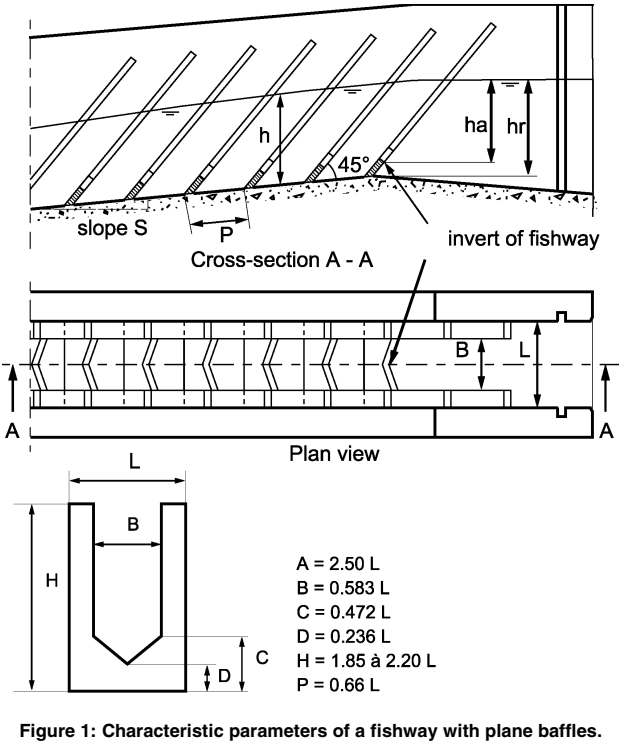# Plane baffles (Denil) fishway

## Geometrical characteristicsExcerpt from Larinier, 20021

## Hydraulic laws given by abacuses

Experiments conducted by Larinier, 20021 allowed to establish abacuses that link adimensional flow $$Q^*$$:

$Q^* = \dfrac{Q}{\sqrt{g}L^{2,5}}$

to upstream head $$ha$$ and the average water level in the pass $$h$$ :Abacuses of a plane baffles (Denil) fishway for a slope of 10% (Excerpt from Larinier, 20021)Abacuses of a plane baffles (Denil) fishway for a slope of 15% (Excerpt from Larinier, 20021)Abacuses of a plane baffles (Denil) fishway for a slope of 20% (Excerpt from Larinier, 20021)

To run calculations for all slopes between 8% and 22%, polynomes coefficients of abacuses above are themelves adjusted in the form of slope $$S$$ depending polynomes.

We thus have:

$ha/L = a_2(S) Q^{*2} + a_1(S) Q^* + a_0(S)$
$a_2(S) = 315.110S^2 - 115.164S + 6.85371$
$a_1(S) = - 184.043S^2 + 59.7073S - 0.530737$
$a_0(S) = 15.2115S^2 - 5.22606S + 0.633654$

And:

$h/L = b_2(S) Q^{*2} + b_1(S) Q^* + b_0$
$b_2(S) = 347.368S^2 - 130.698S + 8.14521$
$b_1(S) = - 139.382S^2 + 47.2186S + 0.0547598$
$b_0(S) = 16.7218S^2 - 6.09624S + 0.834851$

## Calculation of $$ha$$, $$h$$ and $$Q$$

We can then use those coefficients to calculate $$ha$$, $$h$$ and $$Q^*$$:

$ha = L \left( a_2 (Q^*)^2 + a_1 Q^* + a_0 \right)$
$h = L \left( b_2 (Q^*)^2 + b_1 Q^* + b_0 \right)$

Using the positive inverse function, depending on $$ha/L$$, we get:

$Q^* = \dfrac{-a_1 + \sqrt{a_1^2 - 4 a_2 (a_0 - h_a/L)}}{2 a_2}$

And we finally have:

$Q = Q^* \sqrt{g} L^{2,5}$

Calculation limitations of $$Q^*$$, $$ha/L$$ and $$h/L$$ are determined based on the extremities of the abacuses curves.

## Flow velocity

Flow velocity $$V$$ corresponds to the minimum flow speed given the flow section $$A_w$$ at the perpendicular of the baffle :

$V = \dfrac{Q}{A_w}$

for plane baffles fishways using the notation of the schema above, we have:

$A_w = B \times \left( h - \dfrac{C+D}{2} \sin(45°) \right)$

Which gives with standard proportions:

$A_w = L \left(0.583 h - 0.146L \right)$

## Upstream apron elevation $$Z_{r1}$$

$Z_{r1} = Z_{d1} - D \sin(45° + \arctan(S))$

## Minimal rake height of upstream side walls $$Z_m$$

$Z_m = Z_{r1} + - H_{min} \sin(45° + \arctan(S))$

1. Larinier, M. 2002. “BAFFLE FISHWAYS.” Bulletin Français de La Pêche et de La Pisciculture, no. 364: 83–101. doi:10.1051/kmae/2002109